Exercises

# Division 2

In this 5th grade division unit, students will take their understanding to the next level by applying division skills with multi-digit numbers. Students will also begin to explore division with decimals and practice using estimation to check the reasonableness of their answers. Last, students will use their knowledge of the base ten number system to divide decimals by base ten exponents (i.e. .4 ➗ 104).
This lesson includes 5 printable learning activities.

## Division and Powers of Ten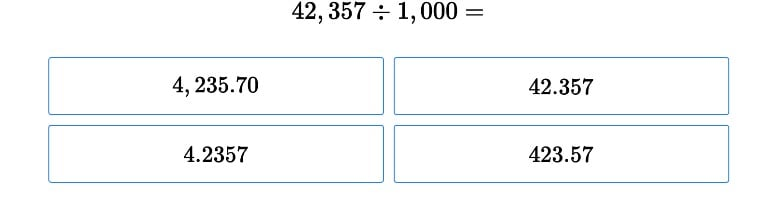Show students how to simplify division problems by drawing on a newfound understanding of the powers of ten.

## Division With Two-Digit Divisors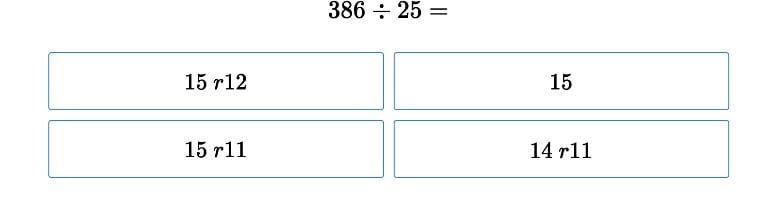When students begin to grow adept at division, expand their skillset by introducing them to two digit divisors.

## Division with Multi-Digit Dividends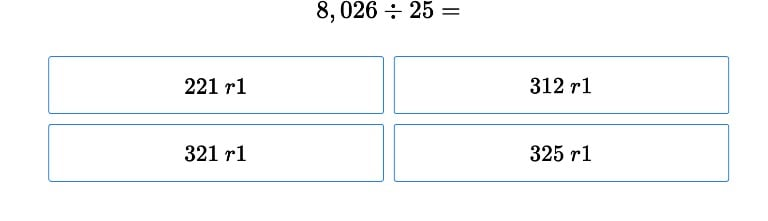Expand students’ division skills with this exercise that teaches how to perform division problems with multi digit dividends.

## Decimal Division with Estimation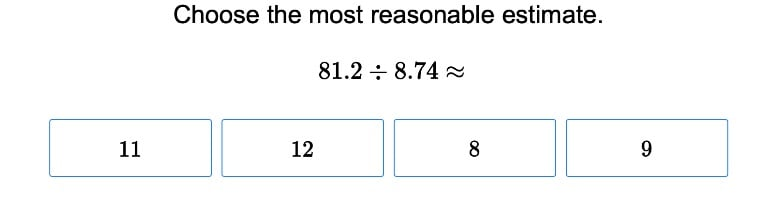When the answer doesn’t need to be precise, students can find the answer to a decimal division problem using estimation.

## Multi-Digit Decimal DivisionOnce students have mastered decimal division to the tenths place, elevate their skills with this exercise on multi digit decimal division.

## Shopping: Decimal Division to the Tenths Place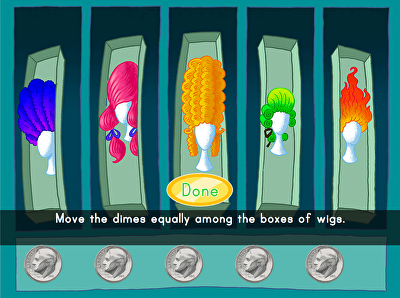It's time to shop 'til you drop! Kids have change in the bank to spend on wigs, cute pets, and new watches in this fun division game. Players must divide their money equally among the three shops, practicing decimal division to the tenth place. After countless rounds of gameplay, kids will have an excellent understanding of where division meets place value.

## Decimal Division with Pennies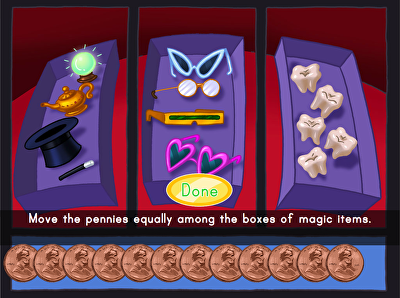Magic items, skate supplies and masks, oh my! Practice division to the hundredths place by shopping in this interactive place value game. Fifth graders will practice moving a decimal point to divide successfully by dividing coins equally among three shops. By moving manipulatives, kids are able to visualize each division problem being solved, helping them master strategies to help them in math.

## Decimal Division to the Hundredths PlaceIn this exercise, students will learn how to execute division problems with decimals to the hundredths place.